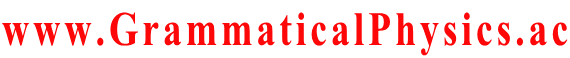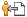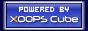Welcome SourceCodeOf_HumanGenome
www.GrammaticalPhysics.ac
 Home News Forum Links Sitemap LogCounter
Account
Access Detector
1 user(s) are online (1 user(s) are browsing Forum)

Members: 1
Guests: 0

SourceCodeOf_HumanGenome more...

# JPS 2007 Spring Meeting

SourceCodeOf_HumanGenome > JPS 2007 Spring Meeting @ 2009/8/4 9:55
28pSL-11
New grammar version of Schrodinger equation
Uda's School / Yuuichi Uda
---
About a system with one degree of freedom, I want to find an equation obeyed by Φ in the new grammar, which represents a quantum history whose quantum state at time t is represented by wave function ψ(□,t) by the following functional Φ.
Φ[χ]=exp[α∫dt φ(χ(t),t)]; ψ(x,t)= exp φ(x,t)・・・※
As a clue, it seems plausible course to select the equation for Φ as it reduces to the ordinary schrodinger equation for ψ in the special case that ※ holds.
Along this course, I tried making the following equation.
(ih／2πα) lim (1／ε)(Φ[χ']－Φ[χ])
ε→0
＝∫dt[(1／2m)(－ih／2πα)^2(δ／δχ(t))^2＋V(χ(t))]Φ[χ],
where χ'(t)=χ(t-ε).
I have not yet confirmed whether this equation always reduces to Schrodinger equation in the special case ※.
Besides, I got some undesirable result when I investigated a solution corresponding to the ground state of a harmonic oscillator.
It is the result that
Φ[χ]＝exp[α∫dt(－π／h)√(mk)(χ(t))^2]
is a solution when
V(x)＝kx^2－(h／4πα)δ(0)√(k／m).
Adding an arbitrary constant to potential energy does not change what physical system we treat, and so it may not be undesirable, but the appearance of the value of δ-function at zero point might mean failure of the theory in the worst case.
In order that V(x)＝kx^2, perhaps we have only to formally select
Φ[χ]＝exp[α∫dt[－Et－(π／h)√(mk)(χ(t))^2]],
using a real constant E.
However, in this case, too, perhaps E includes δ(0), and we should understand that integral ∫dt[－iEt] is not well defined or is zero, and so room for criticism is left.
It might be a way for settling these problems that renew χ'(t)＝χ(t－ε).
For example, how about using an arbitrary function f such that f(t)＝t if t＜a or t＜b, selecting χ'(t)＝χ(f(t)), and altering the Hamiltonian part after it?
In order to avoid being criticized for the point that ψ(x,t) has a dimension of (length)^(-3/2) despite that φ(x,t) is dimensionless, we have only to reselect ψ(x,t)＝βexpφ(x,t) using a dimensionful factor β.
---
Last edited at 2010/05/01/15:33JST

Category
Forum
Global

Notification method is private message [Change]

Site Search
Site Info
WebmasterswooderRecommend Us